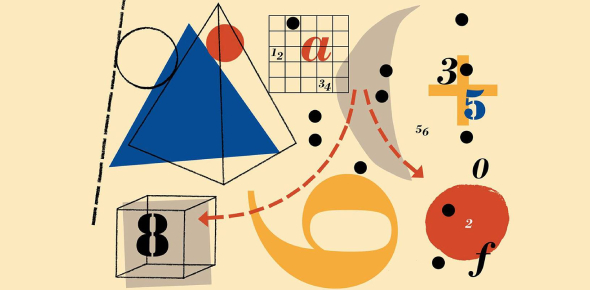# 7th Grade Math: Practice Test! Quiz

13 Questions | Total Attempts: 81SettingsRelated Topics
• 1.
What is the effect of the absolute value on a number?
• A.

The absolute value always makes the number opposite on terms of being positive or negative.

• B.

The absolute value makes any number, whether positive or negative, a positive number

• C.

Absolute value is not an existing term

• D.

The absolute value is any whole number

• 2.
Solve the following equations: 5-(-5)
• A.

10

• B.

-10

• C.

0

• D.

5

• 3.
|-1.50|+1
• A.

-0.50

• B.

1.00

• C.

2.50

• D.

1.50

• 4.
-5*(-5)
• A.

-25

• B.

25

• C.

10

• D.

-10

• 5.
Find all the equivalent fractions in the following question: 1/2
• A.

4/8

• B.

6/10

• C.

50/100

• D.

0.50

• E.

6/3

• 6.
3/4
• A.

75/100

• B.

25/100

• C.

6/8

• D.

5/9

• E.

10/18

• 7.
The following are single questions. Solve the following. 4^2
• A.

16

• B.

-16

• C.

8

• D.

-8

• E.

0

• 8.
0.25 is 1/4 in the form of a decimal.
• A.

True

• B.

False

• 9.
Simplifyj+j+j+j+j+j+j
• A.

8j

• B.

J8

• C.

7

• D.

7j

• 10.
20-5*2
• A.

100

• B.

-10

• C.

78

• D.

10

• 11.
8400100
• 12.
2/0.46
• 13.
7       1016 +   16Write the fraction like this: 2/6